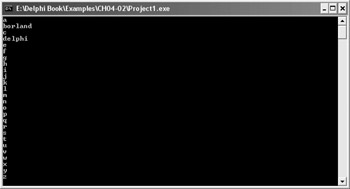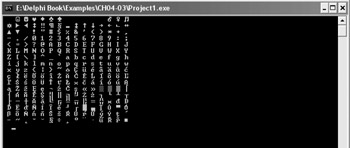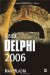Chapter 4: Iterations

This chapter describes three Delphi statements that enable you to repeatedly execute one or more statements. Delphi loops are the for loop, the repeat loop, and the while loop.

The chapter ends with a description of loops in C++.

The for Loop

The for loop is probably the most used of all loop constructs. Unlike other loop constructs, the for loop is used when you already know how many times you have to repeat a statement.

The syntax of the for loop is:

for counter := startValue to endValue do   statement;

The counter is an ordinal (usually integer) value used by the loop to keep track of the number of iterations. startValue and endValue define the total number of iterations. Listing 4-1 shows a basic for loop that displays the user's name several times. You can see the result of the for loop in Figure 4-1.Figure 4-1: Result of the for loop

Listing 4-1: A simple for loopprogram Project1; {\$APPTYPE CONSOLE} uses   SysUtils; var   userName: string;   displayCnt: Integer;   i: Integer; begin   Write('User name: ');   ReadLn(userName);   Write('How many iterations: ');   ReadLn(displayCnt);   WriteLn('----------------------');   for i := 1 to displayCnt do     WriteLn(i, ') ', userName);   ReadLn; end.Although it's pretty easy to write the for loop, the loop itself is pretty complex and a lot of things happen behind the scenes. First, the for loop tries to execute endValue – startValue + 1 iterations. If endValue is less than startValue, the for loop executes nothing. If startValue and endValue are the same, the for loop executes only once. For instance, the following for loop displays the message "Hello":

var   i: Integer; begin   for i := 0 to 0 do     WriteLn('Hello'); end.

If startValue is less than endValue, the loop first executes the statement(s) and then increments the counter variable. When the counter variable reaches the endValue limit, the for loop executes the statement(s) once more and then finishes.

Since the for loop can utilize any ordinal variable as the counter, you can, for instance, use a Char variable to loop through all lowercase letters of the alphabet. You can see the result of this for loop in Figure 4-2.Figure 4-2: Char counter output

Listing 4-2: Char counterprogram Project1; {\$APPTYPE CONSOLE} uses   SysUtils; var   ch: Char; begin   for ch := 'a' to 'z' do   begin     case ch of       'b': WriteLn('borland');       'd': WriteLn('delphi');       else         WriteLn(ch);     end;      // case   end;       // for   ReadLn; end.With a little more code, you can even display the entire ASCII character table using only one for loop. The ASCII (American Standard Code for Information Interchange) table contains numerical values of characters. It defines 256 characters (including letters, punctuation, numbers, and other characters).

Listing 4-3: Displaying the ASCII character tableprogram Project1; {\$APPTYPE CONSOLE} uses   SysUtils; var   ch: Char;   newLine: Integer = 1; begin   { Display the entire ASCII table }   for ch := #0 to #255 do   begin     { Skip CR and LF characters }     if (ch <> #10) and (ch <> #13) then       Write(ch:2);     newLine := newLine + 1;     { Limit to 16 characters per row }     if newLine = 16 then     begin       newLine := 1;       WriteLn;     end;      // if newLine   end;        // for ch   ReadLn; end.Figure 4-3: The ASCII table

The newLine variable is used to limit the number of characters per row to 16. Limiting the number of characters per row without using the newLine variable can be accomplished by typecasting (converting a variable to another data type) the ch variable to an integer. The code that converts a Char variable to an integer variable is listed here, but implicit and explicit typecasting is explained in more detail in Chapter 5.

Listing 4-4: For loop with typecastingprogram Project1; {\$APPTYPE CONSOLE} uses   SysUtils; var   ch: Char; begin   { Display the entire ASCII table }   for ch := #0 to #255 do   begin     case ch of       #10, #13:       else begin         if (ch <> #0) and (Integer(ch) mod 16 = 0) then WriteLn;         Write(ch:2);       end; // else     end;      // case   end;       // for   ReadLn; end.In this example, the if-then test for the CR and LF characters has been replaced with the case statement to illustrate another possible use of the case statement. Since there is no code after the colon where we check for characters #10 and #13, iterations 10 and 13 are skipped. The result of this code is the same as the code in Listing 4-3.

Like the if-then and case statements, the for loop can also be nested. Nested for loops can be very helpful in a number of situations. For instance, you can use a nested for loop to easily display the multiplication table, as shown in Listing 4-5 and Figure 4-4.Figure 4-4: The multiplication table

Listing 4-5: Displaying the multiplication tableprogram Project1; {\$APPTYPE CONSOLE} uses   SysUtils; var   i, j: Integer; begin   WriteLn('Multiplication Table:');   WriteLn;   for i := 1 to 10 do   begin     for j := 1 to 10 do     begin       Write((i * j):4);     end;      // for j     WriteLn;   end;       // for i   ReadLn; end.The for-downto Loop

Another flavor of the for loop is the for-downto loop, where the counter variable is decremented rather than incremented. The syntax of the for-downto loop is:

for counter := startValue downto endValue do   statement;

The for-downto loop fails to execute if endValue is greater than startValue. If both values are the same, the loop executes only once. The for-downto loop can, for instance, be used to implement a countdown.

Listing 4-6: The for-downto loopprogram Project1; {\$APPTYPE CONSOLE} uses   SysUtils; var   i: Integer; begin   for i := 10 downto 1 do   begin     if i > 1  then       WriteLn(i, ' seconds')     else       WriteLn(i, ' second');   end;   WriteLn;   WriteLn('Lift-off');   ReadLn; end.The for-in Loop

The for-in loop is the youngest flavor of the for loop; it was added to the Delphi language in version 2005. The for-in loop is used to iterate through elements in a collection. Its syntax is:

for element in collection do    statement;

The simplest collection supported by the for-in loop is the string type (a collection of characters). The iteration variable (element) used in the for-in loop has to be of the same type as the elements in the collection. Since a string is a collection of Char values, you have to use a Char variable to iterate through a string with the for-in loop. The following example uses the for-in loop to display each character in a string on a separate line.

Listing 4-7: The for-in loopThe for loop (any flavor) is usually the fastest and most straightforward way of iterating through a number of items. But, in certain circumstances, the for loop may not be the best solution because of two basic characteristics: The value of the iteration variable cannot be changed in the loop, and the iteration variable is always incremented or decremented by one.

If you need to change the iteration variable in the loop or change the value of the iteration variable by more than one, you have to use the repeat-until or the while loop.Inside Delphi 2006 (Wordware Delphi Developers Library)
ISBN: 1598220039
EAN: 2147483647
Year: 2004
Pages: 212